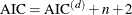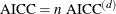### Criteria Used in Model Selection Methods

PROC GLMSELECT supports a variety of fit statistics that you can specify as criteria for the CHOOSE=, SELECT=, and STOP= options in the MODEL statement. The following statistics are available:

adjusted R-square statistic (Darlington, 1968; Judge et al., 1985)

AIC

Akaike’s information criterion (Darlington, 1968; Judge et al., 1985)

AICC

corrected Akaike’s information criterion (Hurvich and Tsai, 1989)

BIC

Sawa Bayesian information criterion (Sawa, 1978; Judge et al., 1985)

CP

Mallows’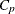statistic (Mallows, 1973; Hocking, 1976)

PRESS

predicted residual sum of squares statistic

SBC

Schwarz Bayesian information criterion (Schwarz, 1978; Judge et al., 1985)

SL

significance level of the F statistic used to assess an effect’s contribution to the fit when it is added to or removed from a model

VALIDATE

average square error over the validation data

Table 45.10 provides formulas and definitions for the fit statistics.

Table 45.10: Formulas and Definitions for Model Fit Summary Statistics

Statistic

Definition or Formula

n

Number of observations

p

Number of parameters including the intercept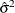Estimate of pure error variance from fitting the full model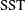Total sum of squares corrected for the mean for the
dependent variable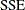Error sum of squares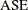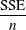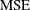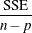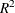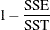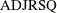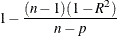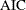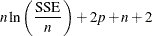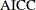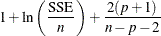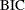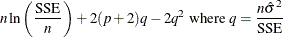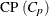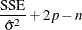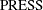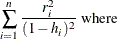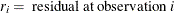and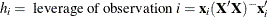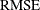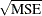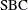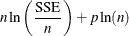#### Formulas for AIC and AICC

There is some inconsistency in the literature on the precise definitions for the AIC and AICC statistics. The definitions used in PROC GLMSELECT changed between the experimental and the production release of the procedure in SAS 9.2. The definitions now used in PROC GLMSELECT yield the same final models as before, but PROC GLMSELECT makes the connection between the AIC statistic and the AICC statistic more transparent.

In the context of linear regression, several different versions of the formulas for AIC and AICC appear in the statistics literature. However, for a fixed number of observations, these different versions differ by additive and positive multiplicative constants. Because the model selected to yield a minimum of a criterion is not affected if the criterion is changed by additive and positive multiplicative constants, these changes in the formula for AIC and AICC do not affect the selection process.

The following section provides details about these changes. Formulas used in the experimental download release are denoted with a superscript of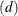and n, p andare defined in Table 45.10.

The experimental download release of PROC GLMSELECT used the following formulas for AIC (Darlington, 1968; Judge et al., 1985) and AICC (Hurvich, Simonoff, and Tsai, 1998):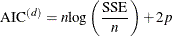and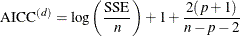PROC GLMSLECT now uses the definitions of AIC and AICC found in Hurvich and Tsai (1989):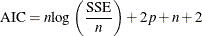and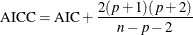Hurvich and Tsai (1989) show that the formula for AICC can also be written as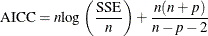The relationships between the alternative forms of the formulas are